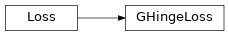GHingeLoss¶

Inheritance Diagramclass ashpy.keras.losses.GHingeLoss[source]

Bases: tensorflow.python.keras.losses.Loss

Generator Hinge Loss as Keras Metric.

See Geometric GAN 1 for more details. The Generator Hinge loss is the hinge version of the adversarial loss. The Hinge loss is defined as:

$L_{\text{hinge}} = \max(0, 1 - t y)$

where y is the Discriminator output and t is the target class (+1 or -1 in the case of binary classification). The target class of the generated images is +1.

For the case of GANs

$L_{G_{\text{hinge}}} = - \mathbb{E}_{(x \sim p_x, y \sim p_data} [ \min(0, -1+D(G(x),y)) ]$

This can be simply approximated as:

$L_{G_{\text{hinge}}} = - \mathbb{E}_{(x \sim p_x, y \sim p_data} [ D(G(x),y) ]$
1

Geometric GAN https://arxiv.org/abs/1705.02894

Methods

 __init__() Initialize the Loss. call(d_real, d_fake) Compute the hinge loss.

Attributes

 reduction Return the current reduction for this type of loss.
__init__()[source]

Initialize the Loss.

Return type

None

call(d_real, d_fake)[source]

Compute the hinge loss.

Return type

Tensor

property reduction

Return the current reduction for this type of loss.

Return type

ReductionV2